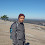### Pearson Correlation using SPSS

Key points to remember about Pearson correlation:

1. Pearson Correlation is used to find out the association between two numeric variables.
2. The scale of measurement of both the dependent as well as independent variables should be ratio scale type.
3. For example, if we want to find out whether the association between age and income is statistically significant, then we can compute Pearson correlation.
4. The range of coefficient of correlation ranges from -1 to (+)1.
5. Value of coefficient=(-1) indicates perfect inverse (negative) linear relationship between dependent and independent variables while the value of +1 indicates perfect positive linear correlation.
6. Value "0" indicates no correlation.
7. Besides the value of correlation coefficient, we should also look at P-values to see whether the correlation (with given coefficient value) is statistically significant.
Steps to compute Pearson Correlation using SPSS
1. Identify the variable pairs of which you want to compute correlation. In the given example, I chose two variables named age and income

2. Note that the variable type should be "numeric" as shown in above picture.
3. Click on analyze, correlate and bivariate.
4. A dialog box appears as shown in the given picture. Drag the variable pair (e.g. age and income) on the box "variables". Chose whether the test is one tailed or two tailed. It depends on your research objective. Also, note that when you check the box "flag significant correlations", it will show extra information in the output if the association is statistically significant.
5. If you want to show the Means and standard deviation of the variables too in addition, you click on options and select the check boxes "Means and Standard Deviations" and "Cross-product deviations and covariances"
6. Click on continue and click on ok, then you see the following output.
Interpretation of the output
7. From the above output you can see the two tables. First table is the descriptive statistics showing the means and standard deviation of the two variables. The second table is about the correlation between two variables. The correlation of the variable with itself is shown as 1 which is not the matter of our interest. Similarly, the correlation between the two variables is shown as 0.335 which is shown in two places (we can use any one of them). The P-value is shown as 0.000 which is of course less than 0.05 and even 0.01. It means there is positive linear correlation between age and income. The table also shows some extra information which we can utilize as per our need.
There is even a summary message below the table that explains that the relationship between age and income from a given example is statistically significant at P<0.01.

The following video shows the short explanation on the steps of calculating pearson correlation using SPSS.

Hope you enjoyed the tutorial. Please comment below for any queries or feedback. Also share if you liked. See you in the next tutorial!

1.well, its informative. and add more statistics using SPSS

1.Thank you so much! I will keep updating the new posts!!

2.Easily understandable even Kindergarden kids. Good work keep it up. if possible upload Logistic Regression.

3.I read this one https://www.learningspss.com/2016/06/multiple-response-analysis-using-spss.html how do I combine this with a multi response question where a respondent can give multiple answers (like in your example)?